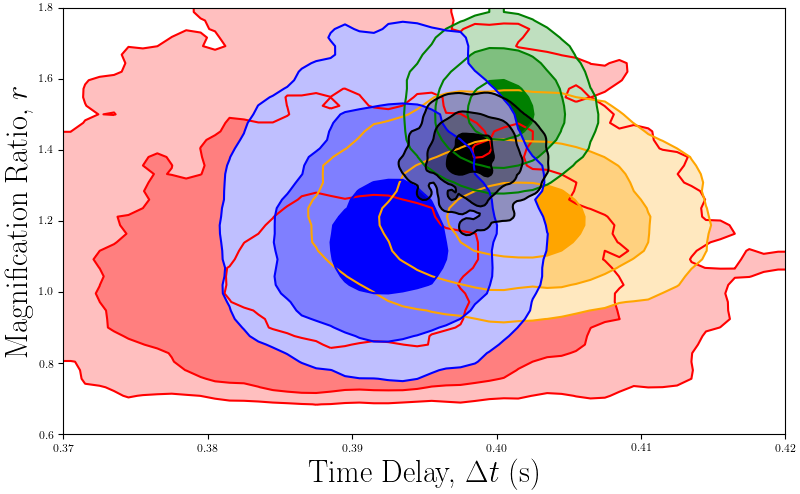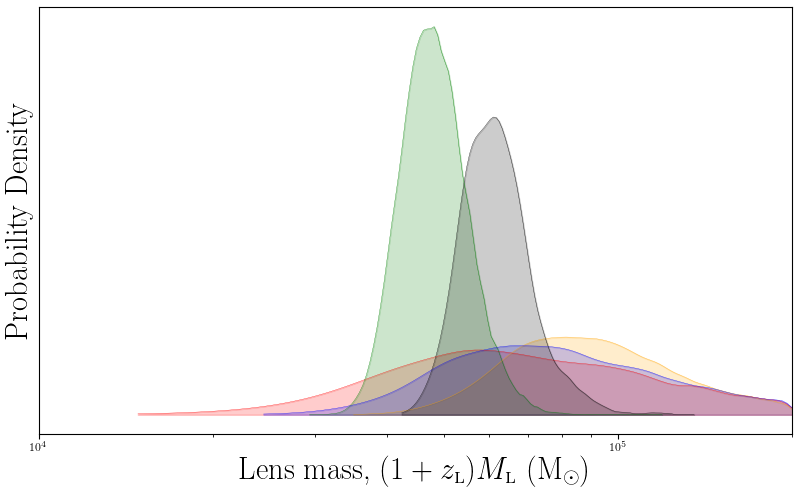# GRB 950830 basic analysis¶

This script compares the two simplest pulse models for a gravitational lensing event for GRB 950830.

gravlensnaturebasic.py
import sys, os
import argparse

from PyGRB.main.fitpulse import PulseFitter
from PyGRB.backend.makemodels import create_model_from_key
from PyGRB.backend.makemodels import make_two_pulse_models

def load_3770(sampler = 'dynesty', nSamples = 100, **kwargs):
test = PulseFitter(3770, times = (-.1, 1),
datatype = 'tte', nSamples = nSamples, sampler = sampler,
priors_pulse_start = -.1, priors_pulse_end = 0.6,
priors_td_lo = 0,  priors_td_hi = 0.5, p_type ='docs', **kwargs)
return test

def evidence_for_3770():
num_samples = 
for samples in num_samples:
GRB = load_3770(sampler = SAMPLER, nSamples = samples)
GRB.offsets = [0, 4000, 8000, -3000]

keys = ['FL', 'FF']
model_dict = {}
for key in keys:
model_dict[key] = create_model_from_key(key)
models = [model for key, model in model_dict.items()]

for model in models:
GRB.main_multi_channel(channels = [0, 1, 2, 3], model = model)
lens_bounds = [(0.37, 0.42), (0.60, 1.8)]
GRB.lens_calc(model = model, lens_bounds = lens_bounds)
GRB.get_evidence_from_models(model_dict = model_dict)

if __name__ == '__main__':
SAMPLER = 'nestle'
evidence_for_3770()


Channel 1

 Model ln Z error ln BF FL -692.95 0.29 3.75 FF -696.70 0.31 0.00

Channel 2

 Model ln Z error ln BF FL -663.86 0.32 6.67 FF -670.53 0.36 0.00

Channel 3

 Model ln Z error ln BF FL -719.66 0.34 5.16 FF -724.82 0.39 0.00

Channel 4

 Model ln Z error ln BF FL -629.50 0.30 0.86 FF -630.36 0.35 0.00

The total ln evidence in favour of lensing is 16.38, which is considered strong evidence. Thus this gamma-ray burst warrants further investigation.BATSE trigger 3770 magnification ratio and time delay posterior histogram.

The magnification ratios and time delays are concordant, as one would expect from a gravitational lensing event.BATSE trigger 3770 lens mass posterior histogram.

The inferred lens mass suggests an intermediate mass black hole as the gravitational deflector.